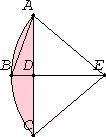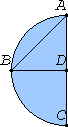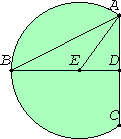# Proposition 25

Given a segment of a circle, to describe the complete circle of which it is a segment.

Let ABC be the given segment of a circle.

It is required to describe the complete circle belonging to the segment ABC, that is, of which it is a segment.

Bisect AC at D, draw DB from the point D at right angles to AC, and join AB.The angle ABD is then greater than, equal to, or less than the angle BAD.

I.23

First let it be greater. Construct the angle BAE on the straight line BA, and at the point A on it, equal to the angle ABD. Draw DB through to E, and join EC.

I.6

Then, since the angle ABE equals the angle BAE, the straight line EB also equals EA.

I.4

And, since AD equals DC, and DE is common, the two sides AD and DE equal the two sides CD and DE respectively, and the angle ADE equals the angle CDE, for each is right, therefore the base AE equals the base CE.But AE was proved equal to BE, therefore be also equals CE. Therefore the three straight lines AE, EB, and EC equal one another.

III.9

Therefore the circle drawn with center E and radius one of the straight lines AE, EB, or EC also passes through the remaining points and has been completed.

Therefore, given a segment of a circle, the complete circle has been described.And it is manifest that the segment ABC is less than a semicircle, because the center E happens to be outside it.

Similarly, even if the angle ABD equals the angle BAD and AD being equal to each of the two BD and DC, the three straight lines DA, DB, and DC will equal one another, D will be the center of the completed circle, and ABC will clearly be a semicircle.

I.23

But, if the angle ABD is less than the angle BAD, and if we construct, on the straight line BA and at the point A on it, an angle equal to the angle ABD, the center will fall on DB within the segment ABC, and the segment ABC will clearly be greater than a semicircle.

Therefore, given a segment of a circle, the complete circle has been described.

Q.E.F.

## Guide

The construction in this proposition is not used in the rest of the Elements.